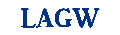Applied Statistics

# APPLIED STATISTICS ASSIGNMENT HELP

Applied Statistics

Statistics was classified in terms of two phases namely Theoretical and Applied Statistics. Theoretical statistics, also called as mathematical statistics, mainly involves the derivation of statistical theorems, development of standard formulas, rules and laws to be used for solving many real-world problems. Applied statistics are mainly deals with the applications of theorems and related concepts developed in theoretical statistics in the area of Business, Research and Development Units, Government Sectors, Marketing Research, Clinical Trials, and so on. According to the theory of statistics, the applied statistics are divided into two categories namely, (i) Descriptive Statistics and (ii) Inferential Statistics.

Descriptive Statistics: Most of the datasets, in its original form, are mostly very large and cannot be directly used for drawing conclusions or making decisions. In order to draw conclusions about such huge data, it is appropriate to calculate descriptive statistics to describe the basic characteristics of the data. The descriptive statistics includes summary tables and graphs/charts, where the summary tables will include certain numerical measures such as mean, median, mode, standard deviation, percentiles and so on, while the graphs/charts includes bar chart, pie chart, histogram, scatter plot, line plot and so on.

Inferential Statistics: If a set of measurements given in the dataset is the entire population, then the conclusion about the dataset has to be drawn only based on the descriptive statistics. However, it not easy to examine the entire population as it is very expensive and time consuming to enumerate the whole population. Due to these reasons, only a small part of the population (usually called a sample) would be considered to answer questions about the whole population. The branch of statistics that deals with making conclusion about the whole population based on the corresponding sample information is called Inferential Statistics. The goal of the inferential statistics is to make inferences about the population characteristics based on the analysis of sample data drawn from this population.

### Applied Statistics Assignment Help

Statisticshelpdesk offers online Applied Statistics assignment help to explore and investigate various theoretical concepts and theorems of theoretical statistics. Our Applied Statistics Assignment help services comprises of all solution to complex problems associated with Applied Statistics. Our step by step approach helps students to understand the solution themselves. We provide Applied Statistics Assignment help through email where a student can quickly upload his Applied Statistics Homework on our website and get it done before the due date.

### Applied Statistics Homework Help

Applied Statistics Homework Help covers all homework and course work questions in Applied Statistics. Our tutors are highly efficient in teaching the use and application of Applied Statistics concepts on various business problems, government and marketing research.. Students can learn to get the best advantage out of Applied Statistics in solving various statistical and managerial problems. Our online Applied Statistics Homework help is a one stop solution to get last minute help in exams, practicals, quizzes and tests.

### Applied Statistics Online Tutor

Our Applied Statistics online tutors are highly experienced statistics tutors with over three years of academic teaching experience as well as research. We provide Applied Statistics Tutor Help online service in which a student can have a direct interaction with our tutors online in the form of live chatting and online session. The student can take the advantage of exam preparation and seek help in his/her quizzes and tests. We provide convenient and easy services at affordable session rates to students seeking help from online Statistics tutor.

SUBMIT HOMEWORK
• Submit your homework for a free quote
• Name:*
• Email:*
• Phone:*
• Subject:*Change Code